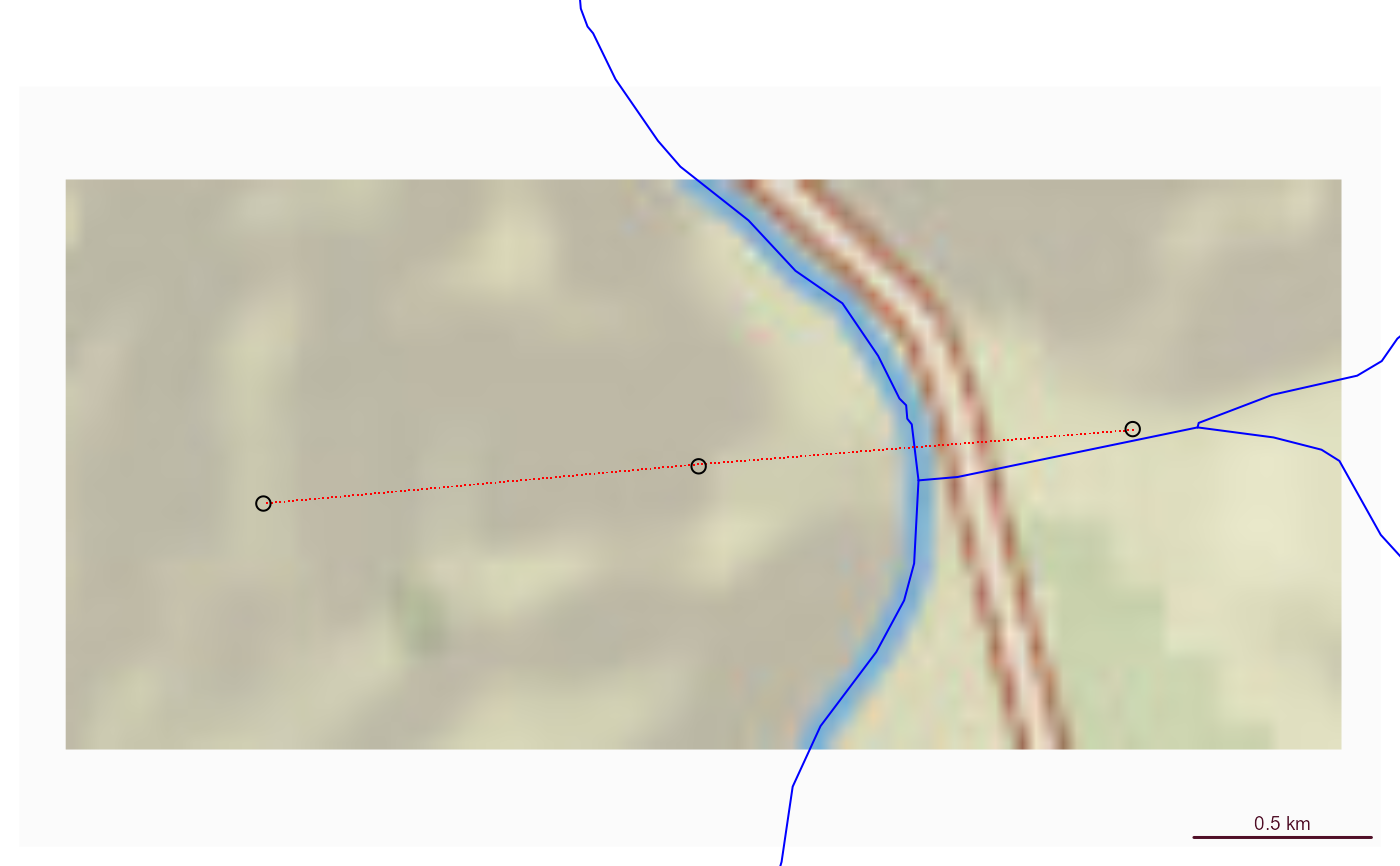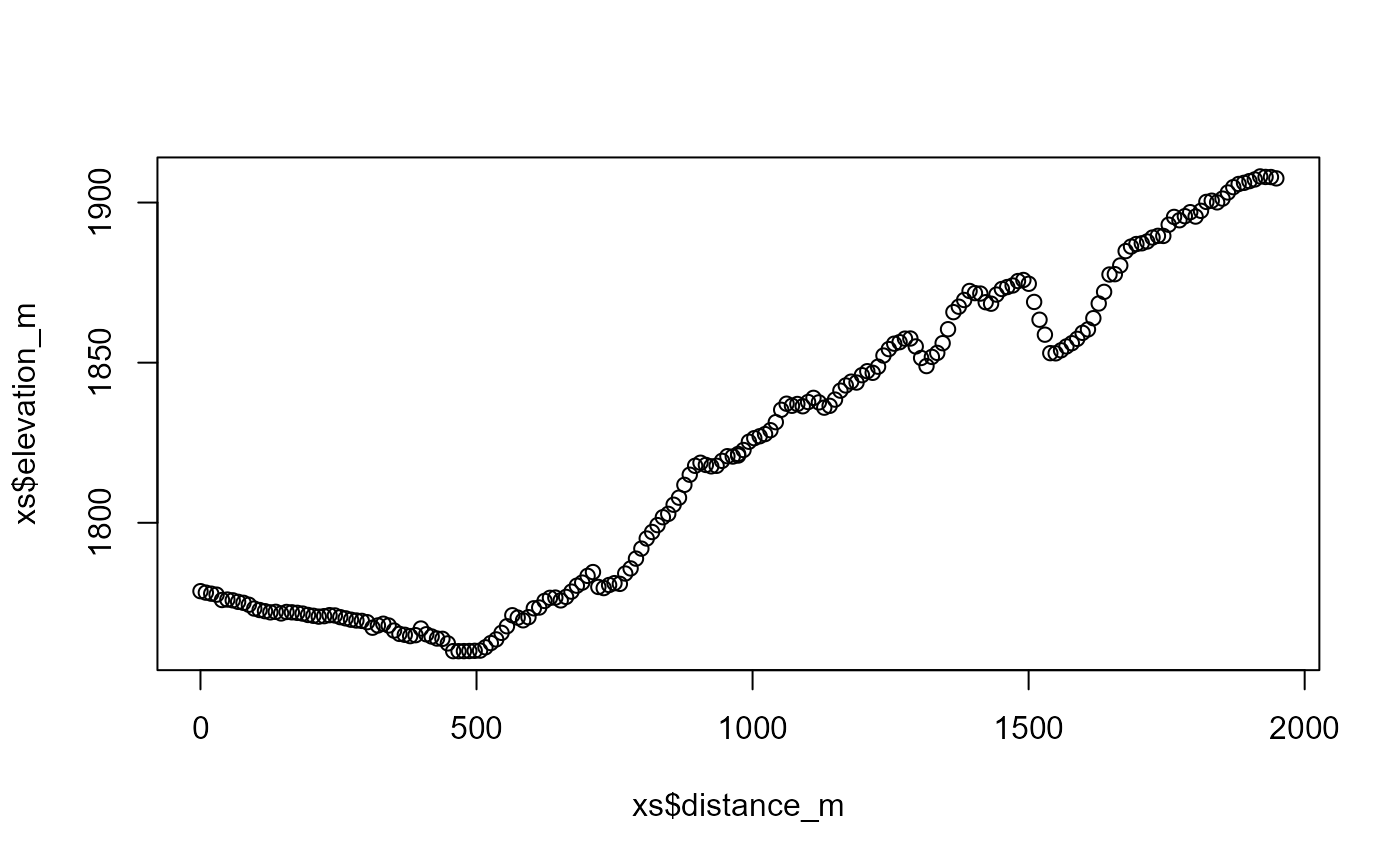Uses a cross section retrieval web services to retrieve elevation along a path.

get_elev_along_path(points, num_pts, res = 1, status = TRUE)

## Arguments

points

sf data.frame containing a point column.

num_pts

numeric number of points to retrieve along the cross section.

res

integer resolution of 3D Elevation Program data to request. Must be on of: 1, 3, 5, 10, 30, 60.

status

logical

## Value

sf data.frame containing points retrieved. Names include "id", "distance_m", "elevation_m", "spatial_ref", "geometry", and ".group". .group tracks which input point each set of output points belongs to.

## Examples

# \donttest{
point1 <- sf::st_sfc(sf::st_point(x = c(-105.9667, 36.17602)), crs = 4326)
point2 <- sf::st_sfc(sf::st_point(x = c(-105.97768, 36.17526)), crs = 4326)
point3 <- sf::st_sfc(sf::st_point(x = c(-105.98869, 36.17450)), crs = 4326)

points <- sf::st_as_sf(c(point1, point2, point3))

(xs <- get_elev_along_path(points, 100))
#> Requestion segment 1 of 2
#> Requestion segment 2 of 2
#> Simple feature collection with 202 features and 5 fields
#> Geometry type: POINT
#> Dimension:     XY
#> Bounding box:  xmin: -105.9886 ymin: 36.17451 xmax: -105.9667 ymax: 36.176
#> Geodetic CRS:  WGS 84
#> # A tibble: 202 × 6
#>    id    spatial_ref elevation_m distance_m             geometry .group
#>  * <chr>       <int>       <dbl>      <dbl>          <POINT [°]>  <int>
#>  1 0               0       1779.       0      (-105.9667 36.176)      1
#>  2 1               0       1778.       9.74 (-105.9668 36.17599)      1
#>  3 2               0       1778.      19.5  (-105.9669 36.17599)      1
#>  4 3               0       1778.      29.2   (-105.967 36.17598)      1
#>  5 4               0       1776.      39.0  (-105.9671 36.17597)      1
#>  6 5               0       1776.      48.7  (-105.9672 36.17597)      1
#>  7 6               0       1776.      58.4  (-105.9674 36.17596)      1
#>  8 7               0       1775.      68.2  (-105.9675 36.17595)      1
#>  9 8               0       1775.      77.9  (-105.9676 36.17594)      1
#> 10 9               0       1774.      87.6  (-105.9677 36.17594)      1
#> # ℹ 192 more rows

if(inherits(xs, "sf")) {

bbox <- sf::st_bbox(xs) + c(-0.005, -0.005, 0.005, 0.005)

nhdplusTools::plot_nhdplus(bbox = bbox, cache_data = FALSE)

plot(sf::st_transform(sf::st_geometry(xs), 3857), pch = ".", add = TRUE, col = "red")
plot(sf::st_transform(sf::st_sfc(point1, crs = 4326), 3857), add = TRUE)
plot(sf::st_transform(sf::st_sfc(point2, crs = 4326), 3857), add = TRUE)
plot(sf::st_transform(sf::st_sfc(point3, crs = 4326), 3857), add = TRUE)

plot(xs$distance_m, xs$elevation_m)
}
#> Spherical geometry (s2) switched off
#> Found invalid geometry, attempting to fix.
#> although coordinates are longitude/latitude, st_intersects assumes that they
#> are planar
#> Spherical geometry (s2) switched on
#> Spherical geometry (s2) switched off
#> although coordinates are longitude/latitude, st_intersects assumes that they
#> are planar
#> Spherical geometry (s2) switched on
#> Spherical geometry (s2) switched off
#> Warning: No nhdarea features found
#> Spherical geometry (s2) switched on
#> Spherical geometry (s2) switched off
#> Warning: No waterbodies features found
#> Spherical geometry (s2) switched on
#> Spherical geometry (s2) switched off
#> Warning: No nonnetwork features found
#> Spherical geometry (s2) switched on
#> Zoom set to: 12# }